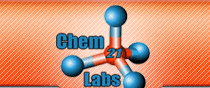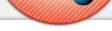Online Lab Submission
Students: Instructors:
Virtual Labs: Pre-Lab Assignments
Interactive Animations:

Unique End-of-Lab Problems

Chem21Labs has the ability to create unique post-lab problems in the following ways . . . .

• Student Numbers (lab data and calculations) stored in the database - any number shown on a student's webpage can be used in a post-lab problem. Use your creativity to challenge students in ways not possible in the paper environment.

You reported a value of 0.0811 L-atm/mole-K for R using the pressureCO2, volumeCO2, temperatureCO2 and massNaHCO3 collected in lab . . . . what mass of NaHCO3 would you need to start with for your experimental R to be 0.08205 L-atm/mole-K if the water vapor pressure was 25.1 torr (assume all the other parameters are the same as reported in your data section)?

• Random Numbers and Arrays
• The Chem21Labs program can generate random numbers with a specific number of significant figures and within a specified range for problems that are algorithmically similar.
• The Chem21Labs program can hold elements of information in arrays. A random number integer can be used to determine which element in the array corresponds to "your question." Both the random number and the array are stored in the database, so you will always get "your question" on page refresh or when logging back in. While there are numerous implementations of this approach, the example below highlights its simplicity. There are 18 images that appear in a post-lab problem . . . . however, a individual student will only have to identify 9 images. A random number (1-2) will determine if a student has to answer Q1 or Q2 . . . . a second random number (3-4) will determine the second answer (Q3 or Q4) . . . . etc. etc. An answer array corresponding to the random number (thermometer,beaker,buret,erlenmeyer flask,filter flask, etc, etc) is used to grade the entered answer. If a student's first random number is 2, then the answer is the 2nd element in the array . . . .  beaker .

Describe what you want and we will do it . . . . we love a challenge.

 1 2 3 4 5 6 7 8 9 10 11 12 13 14 15 16 17 18